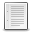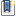File : Details

# mesharea()

Computes the area of any 3D surface mapped with a lattice of 4-vertices cells
Details
Version
1.0
Author
Samuel Gougeon
Maintainer
Samuel Gougeon
Category
Supported Scilab Version
5.5
Creation Date
August 16, 2015Description
```            --> mesharea

Computes the area of any 3D surface mapped with a {X,Y,Z} lattice of 4-vertices
cells.

SYNTAX
------
mesharea  // Displays this help
area = mesharea(X, Y, Z)

PARAMETERS
----------
X,Y,Z : 3 matrices of real decimal numbers, with same sizes:
Cartesian coordinates of the nodes of the lattice mapping the surface.
{X(i,j), Y(i,j), Z(i,j)} locates the node (i,j).
Each node (not on a border or a corner) is linked to 4 neighboors.
Each cell of the lattice has 4 vertices set to nodes.
For a closed surface, the first and last rows of X|Y|Z must be equal,
as well as their first and last columns.
Nan values are accepted. Each node having at least one of its x|y|z
coordinates set to %nan is canceled. This allows for instance to make
holes in the surface.
Folded surfaces are possible, when X, Y, and/or Z are not regular
nor monotonic grids.

area : single positive decimal number. The area of the mesh.
Each 4-vertices cell is split into 2 plan triangles. The area of the
cell is the sum of both triangles areas. The area of the mesh is the
sum of all cells areas.
When a vertice is Nan, the area of the 4 triangles (half cells)
touching it is ignored and not taken into account.

REFERENCE
---------

--------
polygonmetrics : https://fileexchange.scilab.org/toolboxes/445000

EXAMPLE
-------
// Area of a sphere: Study of the error versus the surface sampling
n = 4;
for i = 1:8
long = linspace(0, 2*%pi, 2*n+1);     // sampling longitudes
lat  = linspace(-%pi/2, %pi/2, n+1)'; // sampling latitudes
X = cos(lat)*cos(long);
Y = cos(lat)*sin(long);
Z = sin(lat)*ones(long);
err(i) = mesharea(X, Y, Z)/(4*%pi) - 1;
dAngle(i) = 180/n;  // angular step [°]
n = n*2;
end
clf, plot2d("ll", dAngle, -err)
xtitle('mesharea() accuracy for a sphere', 'Angular sampling [°]', ..
'Relative error on sphere''s area')
// => The relative error goes as the sampling step^2
```Files (2)Screenshot
```Screenshot for thumbnail
```Miscellaneous file
```Macro defining the function mesharea()

* MAKE mesharea() AVAILABLE in EVERY SESSION: Click here to see HOW TO

* Get help: Enter "mesharea" without parameters

```News (0)Comments (0)Leave a comment
Login withEmail notifications